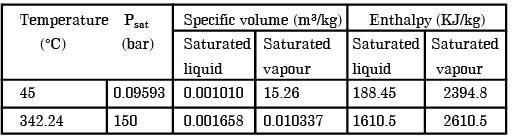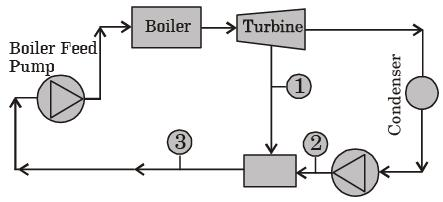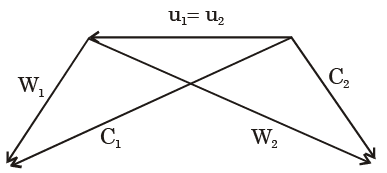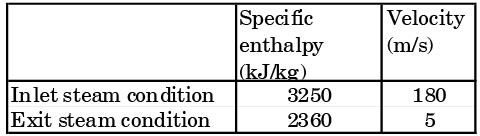Courses

# Past Year Questions: Power Plant Engineering Notes | EduRev

## Mechanical Engineering : Past Year Questions: Power Plant Engineering Notes | EduRev

The document Past Year Questions: Power Plant Engineering Notes | EduRev is a part of the Mechanical Engineering Course Thermodynamics.
All you need of Mechanical Engineering at this link: Mechanical Engineering

Question 1:Boiler rating is usually defined in terms of



Question 2:The equivalent evaporation (kg/hr) of a boiler producing 2000 kg/hr of steam with enthalpy content of 2426 kJ/kg from feed water at temperature 40°C (liquid enthalpy =168 kJ/kg) is (enthalpy of vaporization of water at 100°C = 2258 kJ/kg)



Question 3:Which among the following is the boiler mounting?



Question 4:When initially dry and saturated steam flows through a nozzle, the ratio of actual discharge to calculated discharge is



Question 5:Subsonic and supersonic diffusers have the following geometry.



Question 6:The isentropic heat drop in the nozzle of an impulse steam turbine with a nozzle efficiency 0.9, blade velocity ratio 0.5 and mean blade velocity 150 m/s in kJ/kg is



Question 7:In the Rankine cycle when superheated steam is used



Question 8:In a Rankine cycle heat is added



Question 9:In steam and other vapour cycle, the process of removing non-condensable is called



Question 10:A gas turbine cycle with heat exchange and reheating improves



Question 11:For a given set of operating pressure limits of a Rankine cycle the highest efficiency occurs for



Question 12:A gas turbine cycle with infinitely large number of stages during compression and expansion leads to



Question 13:Consider an actual regenerative Rankine cycle with one open feed water heater. For each kg steam entering the turbine, if m kg steam with a specific enthalpy of h1 is blade from the turbine, and the specific enthalpy of liquid water entering the heater is h2, then h3 the specific enthalpy of saturated liquid leaving the heater is equal to



Question 14:The efficiency of superheat Rankine cycle is higher than that of simple Rankine cycle because



Question 15:In Rankine cycle, regeneration results inhigher efficiency because



Question 16:In a gas turbine, hot combustion products with the specific heats cp = 0.98 kJ/kgK, and cv = 0.7538 kJ/kgK enters the turbine at 20 bar, 1500 K and exits at 1 bar. The isentropic efficiency of the turbine is 0.94. The work developed by the turbine per kg of gas flow is



Question 17:The compression ratio of a gas power plant cycle corresponding to maximum work output for the given temperature limits of Tmin and Tmax will be



Question 18:Given below is an extract from steam tables.​Specific enthalpy of water in kJ/kg at 150 bar and 45°C is



Question 19:Determine the correctness or otherwise Assertion (A) and the Reason (R).
Assertion (A): In a power plant working on a Rankine cycle, the regenerative feed water heating improves the efficiency of the steam turbine.
Reason (R): The regenerative feed water heating raises the average temperature of heat addition in the Rankine cycle.



Question 20:Determine the correctness or otherwise of the following Assertion (A) and the Reason (R).
Assertion (A) : Condenser is an essential equipment in a steam power plant.
Reason (R): For the same mass flow rate and the same pressure rise, a water pump requires substantially less power than a steam compressor.



Question 21:Which combination of the following statements is correct?
The incorporation of reheater in a steam power plant:
P : Always increases the thermal efficiency of the plant.
Q : Always increases the dryness fraction of steam at condenser inlet.
R : Always increases the mean temperature of heat addition.
S : Always increases the specific work output.



Question 22:A thermal power plant operates on a regenerative cycle with a single open feedwater heater, as shown in the figure. For the state points shown, the specific enthalpies are: h1 = 2800 kJ/kg and h2 = 200 kJ/kg. The bleed to the feedwater heater is 20% of the boiler steam generation rate. The specific enthalpy at state 3 isQuestion 23:The values of enthalpy of steam at the inlet and outlet of a steam turbine in a Rankine cycle are 2800 kJ/kg and 1800 kJ/kg respectively.Neglecting pump work, the specific steam consumption in kg/kW hour is



Question 24:An ideal Brayton cycle, operating between the pressure limits of 1 bar and 6 bar, has minimum and maximum temperatures of 300 K and 1500 K. The ratio of specific heats of the working fluid is 1.4. The approximate final temperature in Kelvin at the end of the compression and expansion processes are respectively



Question 25:In a power plant, water (density = 1000 kg/m3) is pumped from 80 kPa to 3 MPa. The pump has an isentropic efficiency of 0.85. Assuming that the temperature of the water remains the same, the specific work (in kJ/kg) supplied to the pump is

[2014,Set-1]

Question 26:The thermal efficiency of an ai r-standard Brayton cycle in terms of pressure ratio rp and y(= cp/cv) is given by

[2014,Set-2]

Question 27:For a gas turbine power plant, identify the correct pair of statements.
P. Smaller in size compared to steam power plant for same power output
Q. Starts quickly compared to steam power plant
R. Works on the principle of Rankine cycle
S. Good compatibility with solid fuel

[2014,Set-3]

Question 28:The INCORRECT statement about regeneration in vapor power cycle is that

[2016,Set-1]

Question 29:Which one of the following modifications of the simple ideal Rankine cycle increases the thermal efficiency and reduces the moisture content of the steam at the turbine outlet?

[2019,Set-2]

Question 30:The current level of the maximum temperature of steam turbine inlet.is much lower than that at gas turbine inlet because



Question 31:For a single stage impulse turbine with rotor diameter of 2 m and a speed of 3000 rpm when the nozzle angle is 20°, the optimum velocity of steam in m/s is



Question 32:A steam power plant has the boiler efficiency of 92%, turbine efficiency (mechanical) of 94%, generator efficiency of 95% and cycle efficiency of 44%. If 6% of the generated power is used to run the auxiliaries, the overall plant efficiency is



Question 33:The following data pertain to a single stage impulse steam turbine:
Nozzle angle = 20°
Relative steam velocity at entry = 350 m/s
If blade friction is neglected the work done per kg steam is



Question 34:If VN and α are the nozzle exit velocity and nozzle angle in an impulse turbine, the optimum blade velocity is given by



Question 35:A Curtis stage, Rateau stage and a 50% reaction stage in a steam turbine are examples of



Question 36:Which of the following is a pressure compounded turbine?



Question 37:The Rateau turbine belongs to the category of



Question 38:Considering the variation of static pressure and absolute velocity in an impulse steam turbine, across one row of moving blades



Question 39:In the velocity diagram shown below, u = blade velocity, C = absolute fluid velocity and W = relative velocity of fluid and the subscripts 1 and 2 refer to inlet and outlet. This diagram is forQuestion 40:Specific enthalpy and velocity of steam at inlet and exit of a steam turbine, running under steady state, are as given below:The rate of heat loss from the turbine per kg of steam flow rate is 5 kW. Neglecting changes in potential energy of steam, the power developed in kW by the steam turbine per kg of steam flow rate, is



Offer running on EduRev: Apply code STAYHOME200 to get INR 200 off on our premium plan EduRev Infinity!

## Thermodynamics

56 videos|92 docs|33 tests

,

,

,

,

,

,

,

,

,

,

,

,

,

,

,

,

,

,

,

,

,

;